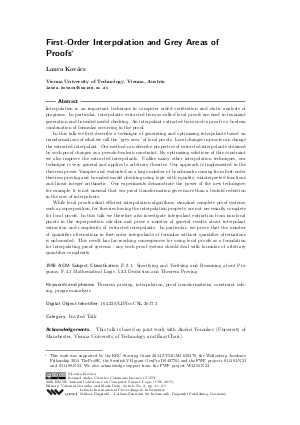Document# First-Order Interpolation and Grey Areas of Proofs (Invited Talk)

### Author Laura Kovács## File

LIPIcs.CSL.2017.3.pdf
• Filesize: 239 kB
• 1 pages

## Cite As

Laura Kovács. First-Order Interpolation and Grey Areas of Proofs (Invited Talk). In 26th EACSL Annual Conference on Computer Science Logic (CSL 2017). Leibniz International Proceedings in Informatics (LIPIcs), Volume 82, p. 3:1, Schloss Dagstuhl - Leibniz-Zentrum für Informatik (2017)
https://doi.org/10.4230/LIPIcs.CSL.2017.3

## Abstract

Interpolation is an important technique in computer aided verification and static analysis of programs. In particular, interpolants extracted from so-called local proofs are used in invariant generation and bounded model checking. An interpolant extracted from such a proof is a boolean combination of formulas occurring in the proof. In this talk we first describe a technique of generating and optimizing interpolants based on transformations of what we call the “grey area” of local proofs. Local changes in proofs can change the extracted interpolant. Our method can describe properties of extracted interpolants obtained by such proof changes as a pseudo-boolean constraint. By optimizing solutions of this constraint we also improve the extracted interpolants. Unlike many other interpolation techniques, our technique is very general and applies to arbitrary theories. Our approach is implemented in the theorem prover Vampire and evaluated on a large number of benchmarks coming from first-order theorem proving and bounded model checking using logic with equality, uninterpreted functions and linear integer arithmetic. Our experiments demonstrate the power of the new techniques: for example, it is not unusual that our proof transformation gives more than a tenfold reduction in the size of interpolants. While local proofs admit efficient interpolation algorithms, standard complete proof systems, such as superposition, for theories having the interpolation property are not necessarily complete for local proofs. In this talk we therefore also investigate interpolant extraction from non-local proofs in the superposition calculus and prove a number of general results about interpolant extraction and complexity of extracted interpolants. In particular, we prove that the number of quantifier alternations in first-order interpolants of formulas without quantifier alternations is unbounded. This result has far-reaching consequences for using local proofs as a foundation for interpolating proof systems - any such proof system should deal with formulas of arbitrary quantifier complexity.
##### Keywords
• theorem proving
• interpolation
• proof transformations
• constraint solving
• program analysis

## Metrics

• Access Statistics
• Total Accesses (updated on a weekly basis)
0